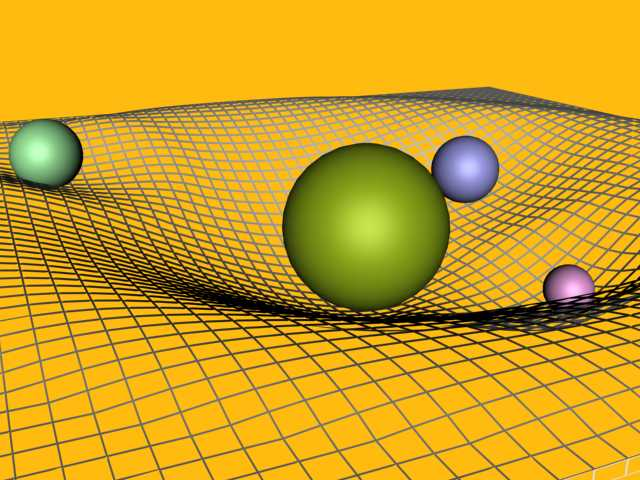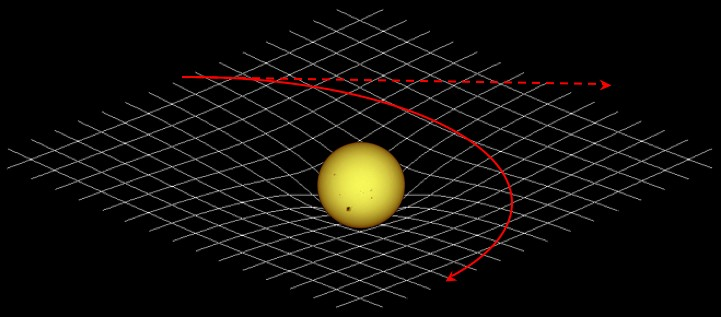In 1915, Einstein showed how to replace gravity with space-time geometry. The mass and energy density of a given region of space determine the geometry, and the path of an object of negligible mass in this space is a geodesic, the shortest possible path.Because this approach is possible only for gravity, it has tended to separate the physics of gravitation entirely from the physics of the other three of the four fundamental forces: the electromagnetic force, the strong force and the weak force. These forces, between 1930 and 1970, were after a long struggle successfully described at the basic quantum level. A satisfactory quantum theory of gravity remains very elusive, to say the least!

All of the novel phenomena predicted by Einstein's theory of gravity have been observed directly, such as gravitational lensing, and the effect of gravity on time, with one exception. Gravitational radiation has yet to be seen directly, although it is confirmed to exist by observing continual energy loss in close binary star systems.

In physics, the word “field” refers to a rule that defines some quantity at every point in space. For example φ(r), if φ is a scalar (number), is called a scalar field. A(r), if A is a vector, is a vector field.

Next